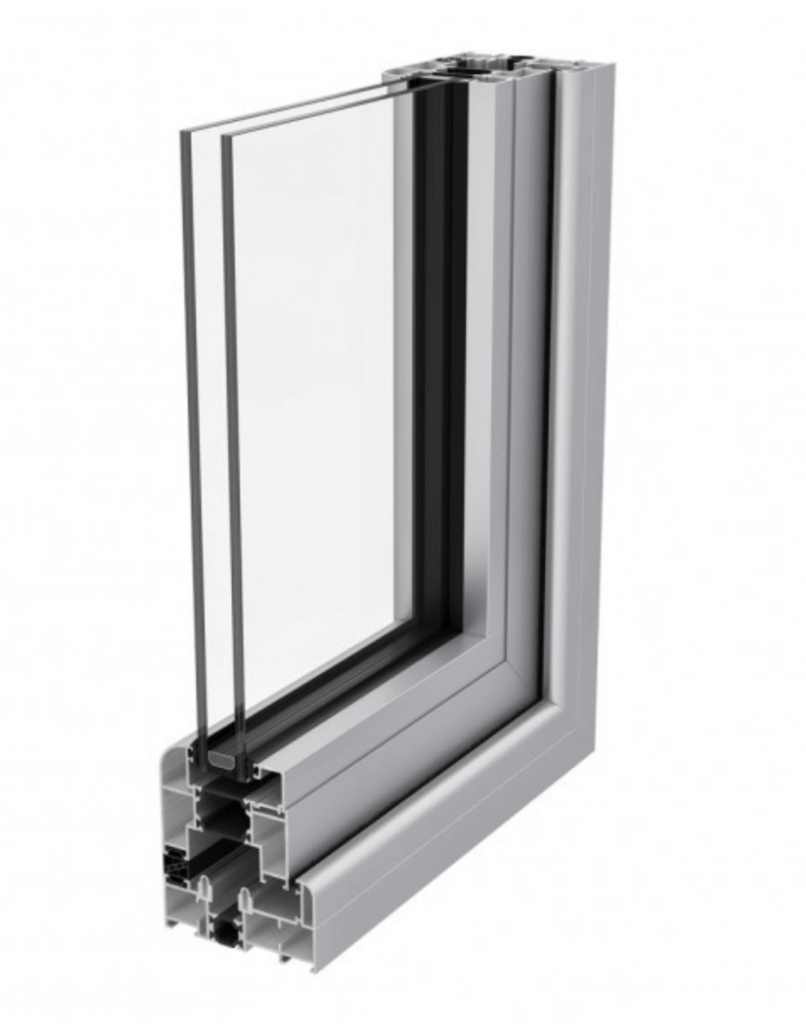# What’s the difference between R-Value and U-value?

U-value and R-value are methods of measuring energy efficiency. They are both used to determine the thermal performance of a building. U-values use a scientific calculation to measure the level of heat transfer through a window. R-value measures the insulating capacity of a specific material and its capacity for heat transfer reduction.

## U-values – essential information

U-value calculates the insulating performance of a window unit. This includes the glass, frame and air gaps. It is used across the glazing industry to determine the energy efficiency performance of windows. U-values are measured in watts per square metre per kelvin (W/m²K). U-value can also be used to:

• Measure thermal efficiency
• Measure insulating capacity
• Indicate heat transfer levels
• Measure airflow
• Measure reflected or radiated heat

U-values are measured using a scientific calculation which determines the rate that heat flows through one square foot of the window. This shows how well a window prevents heat from escaping from a building interior.

## What is a good U-value?U-values are measured on a scale where the lower the number, the better the product at preventing heat loss. Part L of current Building Regulations legislation (mandatory from June 2022) states that a standard window replacement should have a U-value no higher than 1.6 W/m²K for new dwellings and 1.4 W/m²K for existing dwellings. For doors, the required minimum U-value is slightly higher at 1.8 W/m²K.

To provide some context for what a good U-value is, the following information can be helpful to compare materials and their U-values.

 Product/material Average U-value Single glazed window 5.0 W/m²K Double glazed window 1.6 W/m²K Triple glazed window 0.8 W/m²K Solid wall 0.3 W/m²K Roof 0.16 W/m²K Ground floor 0.22 W/m²K

## R-values – essential information

While U-values measure the amount of heat which is transferred through a material, R-values focus on the material’s property value. R-values are often used to find out a material’s insulating capacity. R-values are based on the conduction and resistance of a material to heat transfer. The higher the R-value, the better the material is at insulating and resisting heat transfer. R-values can help us to understand a material’s:

• Energy efficiency performance
• Insulating capacity
• Heat resistance

Although R-values are generally used to understand the thermal performance of different types of building materials, they are also an important part of calculating a window unit’s overall energy efficiency capacity.

## How do R-values affect a window’s energy efficiency rating?

As mentioned above, R-values calculate the thermal performance of a specific material. When assessing a window’s R-value, all material’s performance values must be considered. Whereas U-values only refer to heat transfer through the sealed window unit itself, R-values evaluate the material performance of all other parts of the window including:

• The caulking and sealant used
• The area where the window meets the wall
• Any trims, edging and ancillary components

R-values and U-values are closely linked. If you know the U-value of a window, then you can calculate its R-value and vice versa. To do this the following formulas can be used:

Convert U-value to R-value – divide 1 by U-value eg. U-value = 0.10, 1 ÷ 0.10 = 10

Convert R-value to U-value – divide 1 by R-value eg. R-value = 3.45, 1 ÷ 3.45 = 0.29

These are mathematical reciprocals, and the measurements are determined by the original calculation.

## How can U-values be improved?To ensure that windows meet the required levels for energy efficiency performance, there are several ways to improve a window’s U-value. It’s important to consider U-values in two ways. Summer daytime U-values measure how well a window blocks solar gain to retain an ambient interior temperature. Winter night-time U-values measure a window’s heat retention capacity.

To improve a window’s U-values you can:

• Always specify double or triple glazed Insulated Glass Units (IGUs)
• Used a noble gas such as argon or krypton in the space between the glass panes in the sealed unit
• Increase the size of the space between the glass panes
• Use warm edge spacer bars to create an effective thermal barrier (this is particularly useful in aluminium windows)
• Use low emissivity (Low E) coatings to reduce emissivity and improve reflectance of thermal energy

Understanding the importance of both U-values and R-values is an essential part of window manufacturing and installation. At Exlabesa, we manufacture and supply a range of high performing glazing systems for trade installers.

If you’d like to find out more about any of our products or services, get in touch today. You can call us on 01302 762 500 or send us a message online.